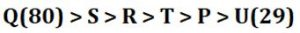Latest Banking jobs   »

# Reasoning Ability Quiz For SBI Clerk Prelims 2022- 20th September

Q1. Dinesh ranked 18th from the top and 24th from the bottom in a class. How many students are there in the class?
(a) 42
(b) 43
(c) 41
(d) 39
(e) 40

Q2. Deepak ranked 28th from the bottom and 15th from the top among those who passed an exam. Nine boys did not participate in the exam and seven failed in it. How many boys were there in the class?
(a) 60
(b) 55
(c) 57
(d) 58
(e) 59

Q3. In a row of boys, Ashu is 18th from the right end and Yatin is 35th from the left end. If in this row Yatin is Fifteenth from the right then what is the position of Ashu from the left?
(a) 31
(b) 32
(c) 30
(d) 33
(e) None of these

Q4. Harsh is 18th from the left end of a row of 37 students and Reena is 18th from the right end in the same row. How many students are there between them in the row?
(a) 2
(b) 3
(c) 5
(d) 1
(e) 4

Q5. Sameer is 20th from the left end of a row and Arun is 12th from the right end of row. If they interchanged their positions, then arun ranks become 10 from right end. Find total number of persons in the row?
(a) 30
(b) 39
(c) 28
(d) 31
(e) None of these

Directions (6-10): Study the following information carefully and answer the given questions.
Amongst Six friends, P, Q R, S, T and U, each got different marks in the examination. T score more marks than U and P. R score more marks than U but less marks than S. P does not score marks that is an odd number. S score marks that are an odd number. S score more marks than T and does not score highest marks. R does not score less than T. The one who scored the minimum marks scored 29 marks and the one who scored the highest, scored 80 marks.

Q6. Who scored the lowest marks?
(a) P
(b) U
(c) S
(d) Q
(e) R

Q7. Who among the following Person has scored third highest marks?
(a) Q
(b) R
(c) T
(d) S
(e) Either (b) or (c)

Q8. If T score 60 marks than what may be the score of R?
(a) 56
(b) 81
(c) 68
(d) 89
(e) 59

Q9. What is possible score of S?
(a) 76
(b) 88
(c) 98
(d) 87
(e) None of these

Q10. Who among the following persons score more than P and less than S?
(a) R
(b) Q
(c) T
(d) Both (a) and (c)
(e) S

Q11. Gopal is 20th from the left end of a row and Geetansh is 25th from the right end of row. If they interchanged their positions then Geetansh ranks become 14 from right end. Find total number of persons in the row?
(a) 32
(b) 35
(c) 34
(d) 33
(e) None of these

Directions (12-14): Study the following information carefully and answer the given questions.
Amongst Six ropes, A, B, C, D, E and F, each has different length. D length is more than E and less than B. A length is more than C and less than B. F length is more than B. C’s length is more than D. The second longest rope length is 16m and third shortest rope length is 9m. D length is 7m.

Q12. Which of the following rope is third longest?
(a) C
(b) B
(c) A
(d) D
(e) Can’t be determined

Q13. What may be the sum of length of E and B?
(a) 23
(b) 24
(c) 21
(d) 25
(e) None of these

Q14. How many ropes are longer than F?
(a) One
(b) Two
(c) Three
(d) None
(e) Can’t be determined

Q15. Harsh is 18th from the left end of a row of 37 students and Reena is 18th from the right end in the same row. How many students are there between them in the row?
(a) 2
(b) 3
(c) 5
(d) 1
(e) 4

Solutions

S1. Ans. (c)
Sol. Number of students in the class = 18+24-1= 41

S2. Ans. (d)
Sol. Number of boys who passed = (28 + 15 – 1) = 42
∴ Total number of boys in the class = (42 + 9 + 7) = 58

S3. Ans. (b)
Sol. Clearly, Ashu is 18th from the right end and
Yatin is 35th from the left end and 15th from the right end of the row
So, number of boys in the row = (35 -1 + 15) = 49
Now, Ashu is 18th from the right
Ashu from left end= 49- (18-1) = 32
Hence, Ashu is 32th from the left end of the row.

S4. Ans. (d)
Sol. Harsh position from right end = (37+1-18) = 20
Students between them = (20-18-1) = 1

S5. Ans. (e)
Sol. Total number of persons in the row = (20+10-1) = 29

Direction (6-10):
Sol.S6. Ans. (b)
S7. Ans. (b)
S8. Ans. (c)
S9. Ans. (e)
S10. Ans. (d)

S11. Ans. (d)
Sol. Total number of persons in the row = (20+14-1) = 33

Solution (12-14):
Sol. The arrangement will be:
F>B(16m)>A>C(9m)>D(7m)>E
S12. Ans. (c)
S13. Ans. (c)
S14. Ans. (d)

S15. Ans. (d)
Sol. Harsh position from right end = (37+1-18) =20
Students between them= (20-18-1) =1#### Congratulations!Download Hindu Review of October 2021: Free PDF